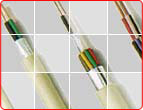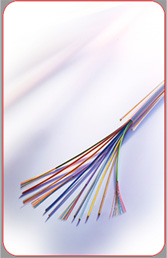Fireproof CablesFire Resistant LSOH Sheathed Cables(fireflix) Fire Retardant LSOH Sheathed Cables(FIRETOX) Flame Retardant PVC Sheathed Cable(FIREGUARD)# FAQ

25.Electrical Terminology Glossary
Amp (A)/Ampere - Amp is a standard unit of current.  The actual quantity of electricity being generated.  The specified amps determine conductor size and type.  In general the larger the conductor the more amps the cable can carry.  The ampere defines the flow rate of electrical current.
Ampacity - Ampacity is the amount of current that a conductor can handle before its temperature exceeds the accepted levels.  Many external factors affect the ampacity of a conductor, such as temperature rating, power loss or heat dissipation.
Annealed - A heat treatment where material is altered causing changes in its properties such as strength and hardness.   Annealing is used to induce ductility, soften material, relieve internal stresses and improve cold working properties.  This process is done by substantially heating the material and then allowing it to cool.  This process is commonly carried out by passing electrical current through the copper wire that heats it to about 700°F.
Attenuation - Attenuation is the loss of signal in communications systems, or loss of electrical power in a length of cable. The magnitude of what goes in one end will be decreased when it comes out the other end.  Losses can occur in the conductor, dielectric (insulation), or the shield.  To reduce the attenuation you can increase the conductor size or lower the dielectric constant.  The less attenuation a cable has the better the cable will perform.  Attenuation is expressed in decibels (dB).
AWG - AWG (gauge) stands for American Wire Gauge.  This is a system for specifying wire size that is predominately used in North America.  The larger the AWG size, the smaller the wire except when the size exceeds #1 AWG, then the larger the number, the larger the wire size.
Capacitance - Capacitance is the property of a system of conductors and dielectrics (insulation) that permits the storage of electrical energy. When a non-conductive material (insulation) is placed between two conductors the insulation will capture some of the signal energy placed on the conductors (like a sponge).  The greater the capacitance, the greater the energy stored.  Generally lower capacitance cable equates to higher performance cable.   For critical signals and/or long cables runs use low-capacitance insulation.  Capacitance is expressed in picofarads per ft. (pf/ft).
Circular Mil (CM) - A term universally used to define cross-sectional areas of conductors.  It is an area equal to the area of a circle 1/1000 of an inch in diameter.  As the number of circular mils increase, the size of a wire increases.  The National Electrical Code (NEC) uses the circular mil to define wire sizes larger than 4/0 AWG. For example a wire size that has a cross section of 250,000 circular mils is typically written as 250 kcmil or 250 MCM.
Conductivity - Capacity of material to carry electrical current, usually expressed as a percentage of copper conductivity, copper being 100%.
Corona - A discharge due to ionization of the air around a conductor due to the potential gradient exceeding a certain critical value. The ions bombard the insulation which in turns breaks molecular bonds and degrades the insulation.
Cross Talk - Cross Talk is a type of interference caused by audio frequencies from one circuit being coupled into an adjacent circuit.  The term is loosely used to also include coupling at high frequencies.  In the simplest terms, cross talk is the undesirable transfer of energy from one cable member to another.  Cross talk happens when signals from one pair of wires interfere with other pairs carrying signals. Have you ever been on the telephone and heard part of another conversation?  This is good example of cross talk.  The twisting of both the conductors and shielding can help reduce cross talk.
Current (I) - Current is the flow of electrons through a conductor.  Current flows when electrons are pushed by the force of an applied voltage.  Current is measured in amperes or amps.  The greater the current the more electrons are moving through the wire at any given time.  If you wish to carry more electrical current, you need a larger diameter of wire.
Ductility - The ability of a conducting material to be drawn out, hammered thin, bent flexed or twisted without breaking.
Flex-life - The measurement of the ability of a conductor or cable to withstand repeated bending.  Flex-life ties into the cable or conductor’s longevity.
Flexibility - The ease with which a cable is bent.
Impedance - Impedance is a material's opposition to the flow of electric current. Similar to Resistance (R), it is measured in ohms (Ω).  Impedance is the resistance to an alternating current along the length of the cable.  It is a relationship between resistance and reactance.  Since it is a relationship, almost every physical characteristic of the cable can affect (e.g., conductor size, insulation thickness, tightness of twisting, etc) it. As a rule of thumb, if a cable's and equipment's impedance has more than a 15% difference - Select a different cable.
Kcmil - This term denotes 1,000 circular mils and replaced "MCM" in the 1990 National Electrical Code (NEC).
Load - Load is a device that consumes or converts the power delivered by another device.  To determine if the load is too heavy use the following equation: W / V = I.  For example if you have a 15 amp circuit and you want to know if your hairdryer will be too heavy a load: 1500 Watts / 120 Volts = 12.5 amps - use your hair dryer.
Ohm (Ω) and Ohm's Law - The Ohm (Ω) is the unit of resistance (R) in a conductor.  Three things determine the amount of resistance in a conductor:
1) Size
2) Conductor Material
3) Temperature
Conductor resistance will increase if the conductor length increases and/or the conductor diameter decreases.
Ohm's Law expresses the relationship between electrical current (I), voltage (V) and resistance (R) in a conductor.  It is the opposition to the flow of current.
Resistance (R) - Resistance (R) is the opposition to electrical current as it passes through a material; most typically a conductor.  Resistance varies depending on the material and size of the conductors being used.  The lower the conductor resistance, the easier the electrons will flow making for a better conductor.  The smaller the conductor, the greater the resistance because the electrical current has a smaller cross - section of material to flow through.  The unit of measure for resistance is the ohm and is represented by the Greek letter Omega (Ω).
Skin Effect - The tendency of alternating current, as its frequency increases to travel only on the surface of the conductor.  As higher frequencies are applied to a conductor the energy is mainly on the "skin" or outer edges of the conductor.
Structural Return Loss (SRL) - Structural Return Loss (SRL) is the measurement of the difference between the energy (signal) sent into the cable and the energy (signal) reflected back.  SRL is measured in decibels (dB).  The higher the number the better the cable.
Tensile Strength - A measure of pull stress required to break any conductor material.
Velocity of Propagation (VP) - Velocity of Propagation (VP) is the speed of transmission of electrical energy in a cable as compared to its speed in air, which is 100% the speed of light.  It is inversely proportional to the dielectric constant.  The lower the dielectric constant, the higher the velocity of propagation.  The VP of a foamed dielectric varies from 78% to 89% whereas that of solid dielectric PE (polyethylene) is 68%.
Voltage (V) - Voltage is the force or "push" that gets the electrons moving through the conductor.  Electrons are very lazy and do nothing unless given some directional push.  Once voltage gets electrons moving they become very productive.  The greater the pressure or push, the greater the capacity to do the work, and the greater the force of electricity being generated.  The pressure is put on free electrons which causes them to flow. The volt is the amount of force required to push a current of one ampere through the conductor with the resistance of one Ohm.
Voltage Standing Wave Ratio (VSWR) - Voltage Standing Wave Ratio (VSWR) is the ratio of the maximum effective voltage to the minimum effective voltage measured along the length of a mismatched radio frequency transmission line.  It is measurement of the efficiency of a cable to transmit power (signal).  A ratio of 1:1 would be a perfect cable.  Standing wave are like echoes on a cable and cause loud and quiet signal levels while an idea cable would have a clearly distinguishable signal.
Watt (W) - A watt is a unit of electrical power. Watts are a measurement of the total amount of electricity being generated.  Simply put watts = amps x volts.

Caledonian Cables |FAQ |© 2019